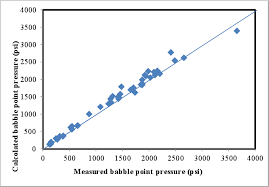## How to Calculate and Solve for Standing Bubble Point | The Calculator EncyclopediaThe image above represents standing bubble point.

To compute for the standing bubble point, three essential parameters are needed and these parameters are Specific Gravity of Solution Gas (γg), Bubble Point Pressure (Pb) and Standing Bubble Point Parameter (a).

The formula for calculating the standing bubble point:

Rs = 18.2[((Pb / γg)0.83 x 10a) – 1.4]

Where:

Rs = Standing Bubble Point
γg = Specific Gravity of Solution Gas
Pb = Bubble Point Pressure
a = Standing Bubble Point Parameter

Let’s solve an example;
Find the standing bubble point with a specific gravity of solution gas of 24, bubble point pressure of 70 and standing bubble point parameter of 45.

This implies that;

γg = Specific Gravity of Solution Gas = 24
Pb = Bubble Point Pressure = 70
a = Standing Bubble Point Parameter = 45

Rs = 18.2[((Pb / γg)0.83 x 10a) – 1.4]
Rs = 18.2[((70 / 24)0.83 x 1045) – 1.4]
Rs = 18.2[((2.916)0.83 x 1045) – 1.4]
Rs = 18.2[(2.43 x 1045) – 1.4]
Rs = 18.2[2.431e+45 – 1.4]
Rs = 18.2[2.431e+45]
Rs = 4.425e+46

Therefore, the standing bubble point is 4.425e+46.